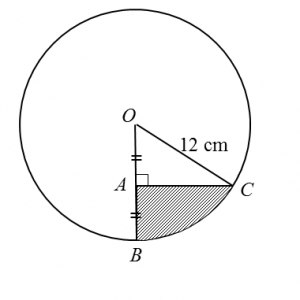# 1.4.2 Circular Measure Short Questions (Question 4, 5 & 6)

Question 4:
Diagram below shows a circle with centre O.The length of the minor arc is 16 cm and the angle of the major sector AOB is 290o.
Using  π = 3.142, find
(b) the length, in cm, of the radius of the circle.

Solution:
(a)
Angle of the minor sector AOB
= 360o 290o
= 70o
= 70o × $\frac{3.142}{180}$

(b)
Using s =
r × 1.222 = 16

Question 5:
Diagram below shows sector OPQ with centre and sector PXY with centre P.Given that OQ = 8 cm, PY = 3 cm ,  ∠ XPY = 1.2 radians and the length of arc PQ = 6cm ,
calculate
( a)  the value of θ , in  radian ,
( b)  the area, in cm2 , of the shaded region .

Solution:
(a) s = θ
6 = 8 θ

(b)
= Area of sector OPQ – Area of sector PXY
$=\frac{1}{2}{\left(8\right)}^{2}\left(0.75\right)-\frac{1}{2}{\left(3\right)}^{2}\left(1.2\right)$
= 24 – 5.4
= 18.6 cm2

Question 6:
Diagram below shows a circle with centre O and radius 12 cm.Given that A, B and C are points such that OA = AB and  ∠OAC = 90°, find
(b)  the area, in cm2, of the shaded region.

Solution:
(a) For triangle OAC,
cos  ∠AOC = 6/12